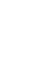Next: Auxiliary utilities Up: MIDAS utilities for time Previous: Time series analysis in

Analysis in the time domain

The commands COVAR/TSA serves for the calculation of the covariance and autocovariance functions. Pairs of signals with matching ACF functions may be analysed further with DELAY/TSA. Matching ACF functions may be obtained for some data after some massaging.

COVAR/TSA -
Covariance analysis: This command computes the discrete covariance function for unevenly sampled data. Edelson and Krolik's (1988) method is used for the estimation of the cross correlation function (CCF) of unevenly sampled series. The binned covariance function is returned with its gaussian errors. Significant are the portions of the curve differing from 0 by more than a number of standard deviations.

This command can also be used for the calculation of the autocovariance function (ACF) by simply using the same series for the two input data sets. Here one shifted series is used as a model for the other. The covariance statistic is used to evaluate the consistency of the two series.

The covariance statistics is akin to the power spectrum statistics and hence to thestatistics (Sect. 12.3.2, Lomb, 1976, Scargle, 1982). The number of degrees of freedom varies among time lag bins. Thus, in order to facilitate the evaluation of the results, errors of the ACF are returned. The expected value of the ACF for pure noise is zero. The value returned for 0 lag corresponds to the correlation of nearby but not identical observations. This is so because the correlation of any observation with itself is ignored in the present algorithm, for numerical reasons. The correlation function for a lag identical to zero can be easily computed as the signal variance.

DELAY/TSA -delay analysis with interpolation: The command computes thetime lag function for two time series by the Press et al. (1992) method. One series is used as a model for the other one, and thestatistics is used to evaluate the consistency of the two series. DELAY/TSA differs from COVAR/TSA in that each series is interpolated to the times of observation in the respective other series. The interpolation is carried out in an elaborate way by using the common autocorrelation function (ACF) of the series. The average value is computed and subtracted from the series so that the resultingis uncorrelated with the average value. This feature of the model enables application to non-stationary series where a mean value is not defined. Because of the interpolation, no coarse binning of the lags is required. Minima of theat a given lag and at a level acceptable for the corresponding number of degrees of freedom indicate a physically significant correlation between the two time series via that lag. The corresponding number of degrees of freedom nr is the number of observations minus the number of fitted parameters (usually 2).

For input, individual measurements must be given with their variances. DELAY/TSA requires the smoothed ACF, common for the two series, to be supplied by the user in analytical form. The form of the ACF can be determined using COVAR/TSA and the MIDAS FIT package (Vol. A, Chapter 8). For this purpose, the ACF of both series should be the same. Often this can be achieved after some massaging of the data. To broaden the ACF, pass the series through a low pass filter. NORMALIZE/TSA may be used to normalize the variances and thus to normalize the ACF maxima. The ACF is passed to the command either via values of the parameters of one of the functions predefined within the TSA package or as the source code of a user-supplied FORTRAN function.

The method is quite new; it should be applied with some caution. Its only presently known practical test has been a consistency check of the results of independent analyses of optical and radio light curves of a pair of gravitationally lensed quasar images (Press et al., 1992). Not only shapes but also values of the ACF should match. This may be achieved by scaling the variances of the observations with NORMALIZE/TSA.Next: Auxiliary utilities Up: MIDAS utilities for time Previous: Time series analysis in
Petra Nass
1999-06-15